Processing ......FreeComputerBooks.com Links to Free Computer, Mathematics, Technical Books all over the World

An Introduction to Bayesian Thinking
Top Free Data Science Books 🌠 - 100% Free or Open Source!
• Title: An Introduction to Bayesian Thinking
• Author(s) Merlise Clyde, Mine Çetinkaya-Rundel, Colin Rundel, David Banks, Christine Chai, Lizzy Huang
• Publisher: GitHub (2021-02-19)
• Paperback: N/A
• eBook: HTML
• Language: English
• ISBN-10/ASIN: N/A
• ISBN-13: N/A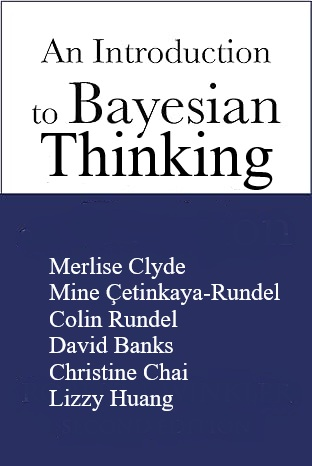Book Description

The goal of this book is to provide an introduction to Bayesian Inference in decision making without requiring calculus. It may be used on its own as an open-access introduction to Bayesian inference using R Programming Language for anyone interested in learning about Bayesian statistics.

The book focuses on Bayesian methods applied routinely in practice including multiple linear regression, mixed effects models and generalized linear models (GLM). The authors include many examples with complete R code and comparisons with analogous frequentist procedures.

• N/A
Reviews, Ratings, and Recommendations: Related Book Categories: Read and Download Links:Similar Books:
•Bayes Rules! An Introduction to Applied Bayesian Modeling

An engaging, sophisticated, and fun introduction to the field of Bayesian statistics, it brings the power of modern Bayesian thinking, modeling, and computing to a broad audience. Integrates R code, including RStan modeling tools, bayesrules package.

•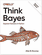O'Reilly® Think Bayes: Bayesian Statistics in Python

If you know how to program, you're ready to tackle Bayesian statistics. With this book, you'll learn how to solve statistical problems with Python code instead of mathematical formulas, using discrete probability distributions rather than continuous mathematics.

•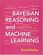Bayesian Reasoning and Machine Learning (David Barber)

This practical introduction is ideally suited to computer scientists without a background in calculus and linear algebra. You'll develop analytical and problem-solving skills that equip them for the real world. Numerous examples and exercises are provided.

•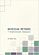Bayesian Methods for Statistical Analysis (Borek Puza)

Bayesian methods for statistical analysis is a book on statistical methods for analysing a wide variety of data. It contains many exercises, all with worked solutions, including complete computer code.

•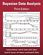Bayesian Data Analysis (Andrew Gelman, et al.)

This classic book is widely considered the leading text on Bayesian methods, lauded for its accessible, practical approach to analyzing data and solving research problems. It takes an applied approach to analysis using up-to-date Bayesian methods.

•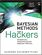Bayesian Methods for Hackers: Probabilistic Programming

This book illuminates Bayesian inference through probabilistic programming with the powerful PyMC language and the closely related Python tools NumPy, SciPy, Matplotlib, through practical examples and computation - no advanced mathematics required.

•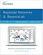Bayesian Networks and BayesiaLab (Stefan Conrady, et al.)

This practical introduction is geared towards scientists who wish to employ Bayesian Networks for applied research using the BayesiaLab software platform. It can serve as a self-study guide for learners and as a reference manual for advanced practitioners.

•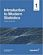Introduction to Modern Statistics (Mine Çetinkaya-Rundel, et al.)

This book puts a heavy emphasis on exploratory data analysis and provides a thorough discussion of simulation-based inference using randomization and bootstrapping, followed by a presentation of the related Central Limit Theorem based approaches.

•Foundations in Statistical Reasoning (Pete Kaslik)

This book is designed for students taking an introductory statistics class. The emphasis throughout the entire book is on how to make decisions with only partial evidence. It focuses on the thought process.

•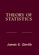Theory of Statistics (James E. Gentle)

This book is directed toward students for whom mathematical statistics is or will become an important part of their lives. It covers classical likelihood, Bayesian, and permutation inference; an introduction to basic asymptotic distribution theory; and modern topics.

Book Categories
 :All CategoriesTop Free BooksRecent BooksMiscellaneous BooksComputer EngineeringComputer LanguagesComputer ScienceData Science/DatabasesJava and Java EE (J2EE)Linux and UnixMathematicsMicrosoft and .NETMobile ComputingNetworking and CommunicationsSoftware EngineeringSpecial TopicsWeb Programming
Other Categories